# Exponential Functions

Go back to  'Functions'

We have discussed exponents and exponential terms in detail in an earlier chapter. If the exponent in an exponential term is variable, we have an exponential function. Consider the function

$f\left( x \right) = {2^x}$

On the right side, the base of the exponential term is fixed, but the exponent is variable. As the exponent is varied, the function output will change. For example:

\begin{align}x = 0\,\,\, \qquad& \Rightarrow\,\,\,f\left( 0 \right) = {2^0} = 1\\x = - 1 \qquad& \Rightarrow\,\,\,f\left( { - 1} \right) = {2^{ - 1}} = \frac{1}{2}\\x = - 10 \qquad& \Rightarrow\,\,\,f\left( { - 10} \right) = {2^{ - 10}} = \frac{1}{{{2^{10}}}}\\x = \sqrt 2 \qquad& \Rightarrow \,\,\,f\left( {\sqrt 2 } \right) = {2^{\sqrt 2 }}\end{align}

What is the domain of f? We have seen earlier that the exponent can take on any real value –positive, negative or zero. In other words, the term $${2^x}$$ has a well-defined meaning for every real value of x. This means that the domain of f is $$\mathbb{R}$$.

What is the range? Firstly, we make the observation that the input set – the domain – is a continuous interval.Also, intuitively, it might seem apparent to you that the function output will not have abrupt jumps. For example, as x is continuously increased from a value $$- 1$$  to 3, should continuously increase from $$\frac{1}{2}$$ to 8. The fact that the output of f will vary continuously can be proved; for now, we will take its truth on face value.

Now, let us try to analyze the behavior of this function in more detail.

Suppose that x is increased in value, starting from 0. How does f behave? In other words, what is the trend followed by the function output? The following table will help us understand this (we have only taken some integer values for x because will be easier to evaluate for integer values – this does not mean that is not defined for non-integer values):

 x $$f\left( x \right)$$ 0 $${2^0} = 1$$ 1 $${2^{ 1}} = 2$$ 2 $${2^{ 2}} = 4$$ 3 $${2^{ 3}} =8$$ 5 $${2^{ 5}} =32$$ 10 $${2^{ 10}} = 1024$$

The trend is clear: as increases, f increases. In fact, f increases really fast! From this data, we can extrapolate that as $$x \to\infty$$, $$f \to \infty$$.

Now, let us see what happens as is decreased, starting from 0 (once again, for ease of calculations, we have taken only integer values of x):

 x $$f\left( x \right)$$ 0 $${2^0} = 1$$ $$- 1$$ $${2^{ - 1}} = \frac{1}{2} = 0.5$$ $$- 2$$ $${2^{ - 2}} = \frac{1}{4} = 0.25$$ $$- 3$$ $${2^{ - 3}} = \frac{1}{8} = 0.125$$ $$- 5$$ $${2^{ - 5}} = \frac{1}{{32}} \approx 0.03$$ $$- 10$$ $${2^{ - 10}} = \frac{1}{{1024}} \approx 0.001$$

The trend is once again clear: as decreases from 0 towards negative infinity, f decreases from 1 towards 0. We can say that as $$x \to - \infty$$,$$f \to 0$$. Note that no matter how large a negative value x takes, f will never become exactly 0,though it will keep coming closer and closer to 0.

The following figure shows the graph of $$f\left( x \right) = {2^x}$$ plotted using a plotter: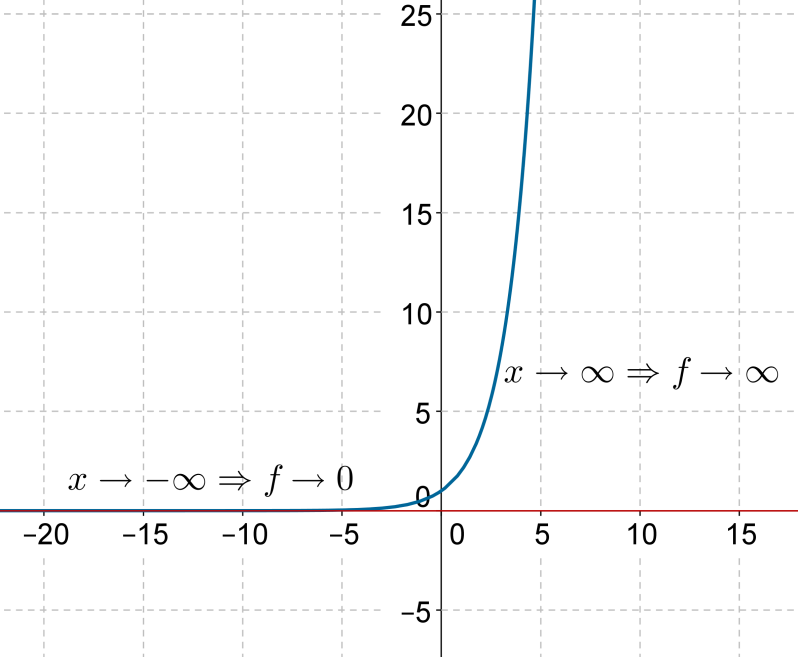Note the spectacular rate of increase towards the positive side. This is understandable: for every unit change in x,the function value doubles. For example, in going from  $$x = 5$$  to$$x = 6$$ , the input variable changes by only 1 unit, but the output changes by 32. On the negative side, note the slow decay towards zero. This is shown in more detail in the following figure: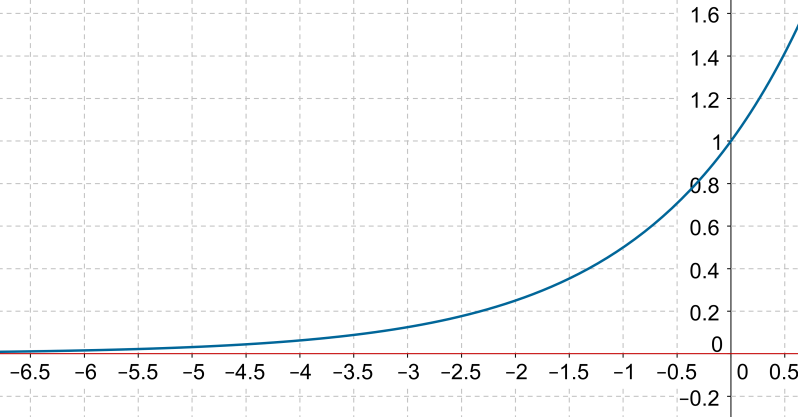The curve never actually touches the x-axis,but it does keep on coming closer and closer towards the x-axis as we move towards negative infinity.

Clearly, the range of $$f\left( x \right) = {2^x}$$ is $$\left( {0,\infty } \right)$$. The number 0 is not included in the range as f never actually becomes 0.

The following figure shows the graph of the function $$g\left( x \right) = {\left({\frac{1}{2}} \right)^x}$$: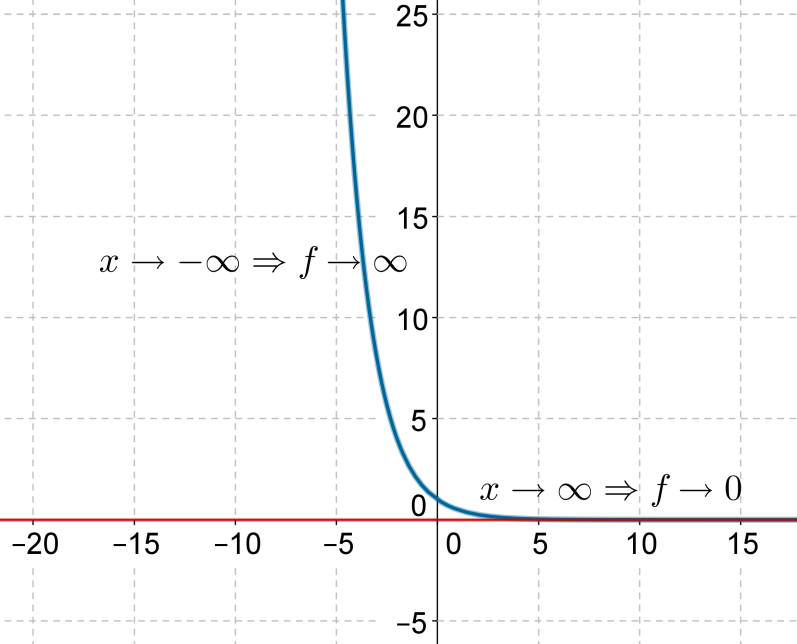It is left to you as an exercise to explain this behavior. The domain and range of g are $$\mathbb{R}$$ and $$\left({0,\infty } \right)$$ again.

What effect does the base have on the behavior of the exponential function? The following figure shows the plots of the functions $${f_1}\left( x \right) = {\left({1.5} \right)^x}$$, $${f_2}\left( x\right) = {2^x}$$ and $${f_3}\left( x\right) = {\left( {2.5} \right)^x}$$ on the same axes: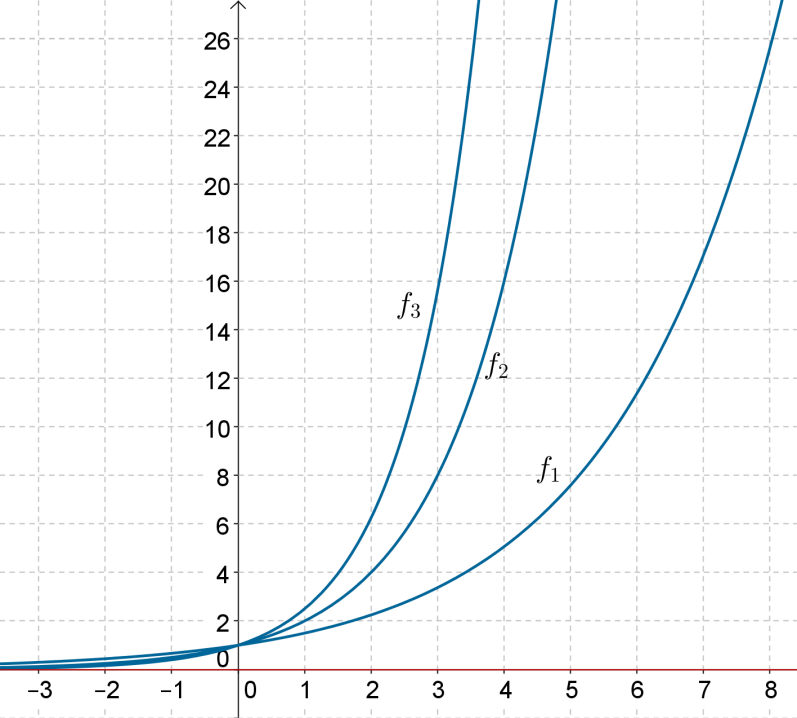The behavior is similar, but the rates of increase are different. For example, the third function increases at a much faster rate than the first function. This is because the base of the third function (2.5) is larger than that of the first (1.5).

Analogously, if the base of the function is between 0 and 1, then the rate of decrease of the function will depend upon how small the base is. As the base gets smaller, the function decreases at faster rates. The following figure shows the plots of the functions $${f_1}\left( x \right) = {\left({\frac{1}{2}} \right)^x}$$, $${f_2}\left(x \right) = {\left( {\frac{1}{3}} \right)^x}$$ and $${f_3}\left( x \right) = {\left( {\frac{1}{4}} \right)^x}$$on the same axes: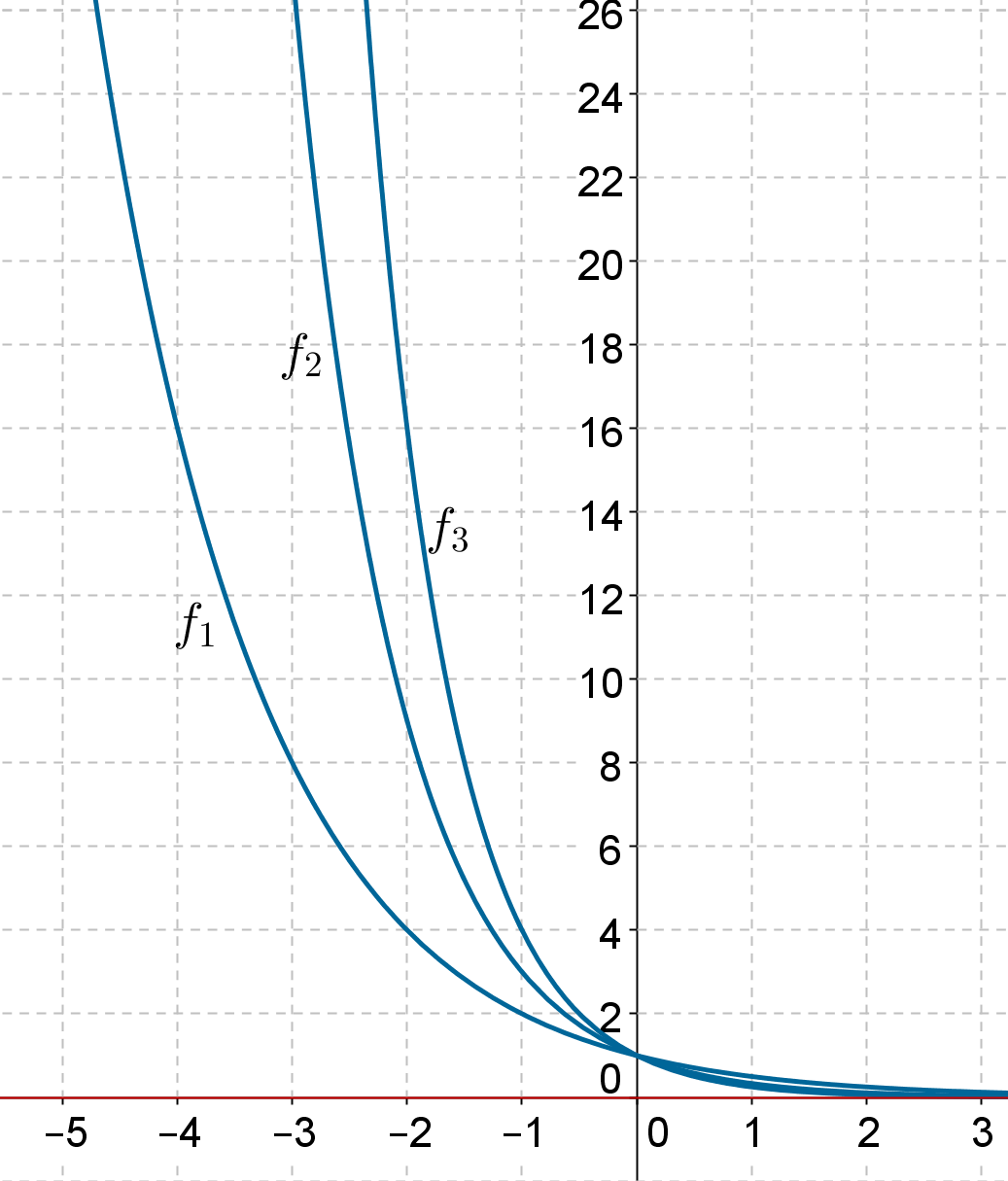Example 1: Find the domain of the function

$f\left( x \right) = \sqrt {{2^x} - 4}$

Solution: The expression under the square-root sign must be non-negative, and so:

$\begin{array}{l}{2^x} - 4 \ge 0\\ \Rightarrow \,\,\,{2^x} \ge 4\,\,\,\Rightarrow \,\,\,{2^x} \ge {2^2}\,\,\, \Rightarrow \,\,\,x \ge 2\\ \Rightarrow \,\,\,D = \left[{2,\infty } \right)\end{array}$

Refer to the graph of $$g\left( x \right) = {2^x}$$ plotted earlier,to understand why $${2^x}$$ will be greater than 4 if x is greater than 2.

Functions
Functions
grade 10 | Questions Set 2
Functions
Functions
grade 10 | Questions Set 1